## A number between 10 and 100 is 8 times the sum of its digits. If 45 is substracted from it, the result equals the number formed by int

Question

A number between 10 and 100 is 8 times the sum of its digits. If 45 is substracted
from it, the result equals the number formed by interchanging its digit. Find the
number

in progress 0
5 months 2021-08-09T15:00:23+00:00 1 Answers 8 views 0

72

Step-by-step explanation:

Let the number be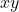Value of number =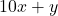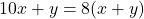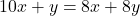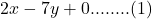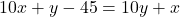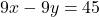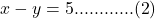Multiply by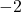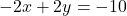Add to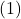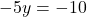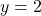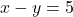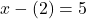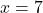Number = 72

hope this helps…